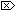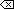The Open Group Base Specifications Issue 6
IEEE Std 1003.1-2001

#### NAME

ilogb, ilogbf, ilogbl - return an unbiased exponent

#### SYNOPSIS

```#include <math.h> int ilogb(double x); int ilogbf(float x); int ilogbl(long double x); ```

#### DESCRIPTION

[CX]The functionality described on this reference page is aligned with the ISO C standard. Any conflict between the requirements described here and the ISO C standard is unintentional. This volume of IEEE Std 1003.1-2001 defers to the ISO C standard.These functions shall return the exponent part of their argument x. Formally, the return value is the integral part of logr|x| as a signed integral value, for non-zero x, where r is the radix of the machine's floating-point arithmetic, which is the value of FLT_RADIX defined in <float.h>.

An application wishing to check for error situations should set errno to zero and call feclearexcept(FE_ALL_EXCEPT) before calling these functions. On return, if errno is non-zero or fetestexcept(FE_INVALID | FE_DIVBYZERO | FE_OVERFLOW | FE_UNDERFLOW) is non-zero, an error has occurred.

#### RETURN VALUE

Upon successful completion, these functions shall return the exponent part of x as a signed integer value. They are equivalent to calling the corresponding logb() function and casting the returned value to type int.

If x is 0, [XSI]a domain error shall occur, andthe value FP_ILOGB0 shall be returned.

If x is ±Inf, [XSI]a domain error shall occur, andthe value {INT_MAX} shall be returned.

If x is a NaN, [XSI]a domain error shall occur, andthe value FP_ILOGBNAN shall be returned.

[XSI]If the correct value is greater than {INT_MAX}, {INT_MAX} shall be returned and a domain error shall occur.

If the correct value is less than {INT_MIN}, {INT_MIN} shall be returned and a domain error shall occur.#### ERRORS

These functions shall fail if:

Domain Error
[XSI]The x argument is zero, NaN, or ±Inf, or the correct value is not representable as an integer.

If the integer expression (math_errhandling & MATH_ERRNO) is non-zero, then errno shall be set to [EDOM]. If the integer expression (math_errhandling & MATH_ERREXCEPT) is non-zero, then the invalid floating-point exception shall be raised.The following sections are informative.

None.

#### APPLICATION USAGE

On error, the expressions (math_errhandling & MATH_ERRNO) and (math_errhandling & MATH_ERREXCEPT) are independent of each other, but at least one of them must be non-zero.

#### RATIONALE

The errors come from taking the expected floating-point value and converting it to int, which is an invalid operation in IEEE Std 754-1985 (since overflow, infinity, and NaN are not representable in a type int), so should be a domain error.

There are no known implementations that overflow. For overflow to happen, {INT_MAX} must be less than LDBL_MAX_EXP*log2(FLT_RADIX) or {INT_MIN} must be greater than LDBL_MIN_EXP*log2(FLT_RADIX) if subnormals are not supported, or {INT_MIN} must be greater than (LDBL_MIN_EXP-LDBL_MANT_DIG)*log2(FLT_RADIX) if subnormals are supported.

#### FUTURE DIRECTIONS

None.

feclearexcept() , fetestexcept() , logb() , scalb() , the Base Definitions volume of IEEE Std 1003.1-2001, Section 4.18, Treatment of Error Conditions for Mathematical Functions, <float.h>, <math.h>

#### CHANGE HISTORY

First released in Issue 4, Version 2.

#### Issue 5

Moved from X/OPEN UNIX extension to BASE.

#### Issue 6

The ilogb() function is no longer marked as an extension.

The ilogbf() and ilogbl() functions are added for alignment with the ISO/IEC 9899:1999 standard.

The RETURN VALUE section is revised for alignment with the ISO/IEC 9899:1999 standard.

XSI extensions are marked.

End of informative text.

UNIX ® is a registered Trademark of The Open Group.
POSIX ® is a registered Trademark of The IEEE.
[ Main Index | XBD | XCU | XSH | XRAT ]